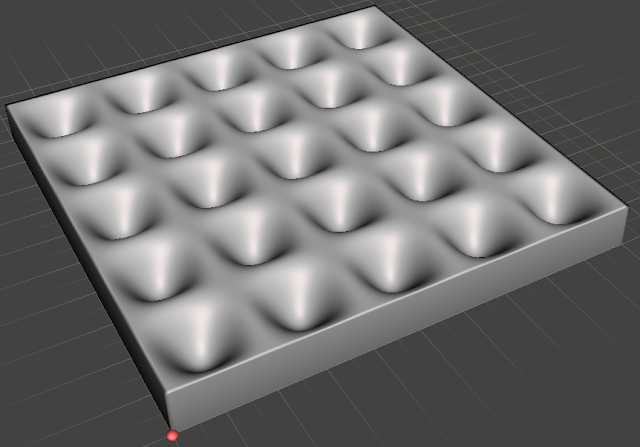## Wednesday, October 2, 2019

### I hope you find this blog enjoyable and helpful. Support it with a small donation.

I recently needed to generate a 3D model for printing based on an equation and couldn't find software to do the job.  In the end the easiest thing to do was to write a quick Python script called "stl-surface.py" to do the job.

At first the task seemed daunting, but generating the STL file is rather easy with the use of the numpy-stl library. The equation for the surface is calculated over a structured square/rectangular grid and each little cell of the grid is split in two to form triangles. You use many many of these small triangular faces to construct the model. All you have to do is to generate the coordinates for the 3 vertices that make up each triangle and numpy-stl does the rest. The bottom and sides are flat which makes things rather easy, and the top is defined by the equation.

When constructing the model it's important to list the coordinates of each face in a counter clockwise direction when looking at the model from the outside. This allows numpy-stl to later calculate things like volume of the model and centre of gravity and also test if the surface is closed (although they use a slightly buggy way to test this).

I've shown a few examples of the generated models below.

10 - 2*(1 - math.cos(2 * x * math.pi / 20)) * (1 - math.cos(2 * y * math.pi / 20))Dimpled 3D model
10 + 2 * math.cos(math.sqrt((x - 50)**2 + (y - 50)**2)/2)Rippled 3D model

Here you can see how the triangles are assembled to construct the model.The triangular faces that make up the model

The triangles that form the edges of the modelEdge of the model

From the top you can see the grid points where the surface function is evaluated.Top of the model

I hope this code can help someone else. I may not be the easiest thing to use and if you need help getting started get in touch.Get the code!

### Support me and the blog with a small donation. I appreciate each one.

1.Hello,
I started working on simulation over rough surfaces recently. To create the rough surface, I needed exactly what you did in this post. I'm relatively new to programming, so I don't understand everything.
One Problem I was running into, is the resolution of the stl file.
Is there a way to make the resolution better without increasing the overall size of the model?

No matter if you have an answer to my question, your code helped me a lot.
Thank you!!

Best regards

2.I keep getting the following error

---------------------------------------------------------------------------
KeyError Traceback (most recent call last)
~\AppData\Local\Temp\ipykernel_1016\668448970.py in
5 # Add faces for the top surface by adding the coordinates of three
6 # vertices to a tuple
----> 7 top_faces[counter * 2 + 1] = ((top_vertices[x_index, y_index],
8 top_vertices[x_index + 1, y_index + 1],
9 top_vertices[x_index, y_index + 1]))

KeyError: (0, 0)